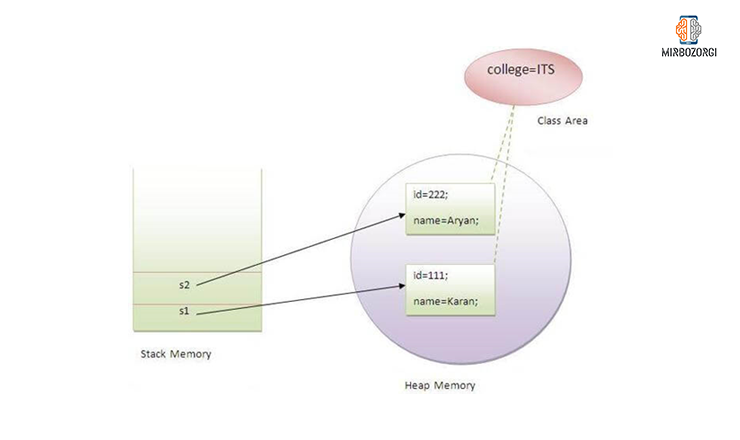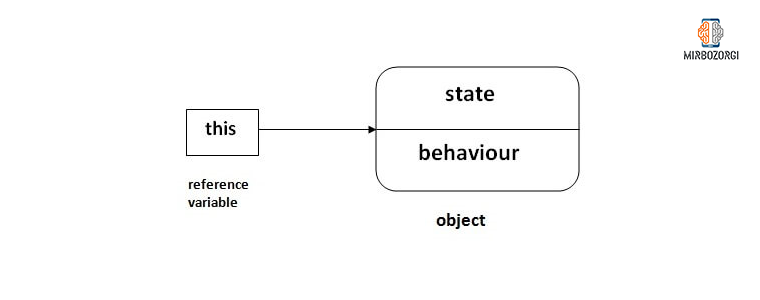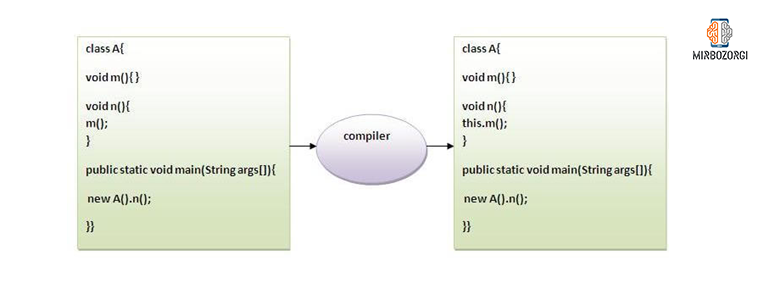## Static and This:

My name is Arsalan Mirbozorgi and in this article, I am trying to talk to you about two keywords in Java. There are so many different types of keywords in Java that you cannot use them to name variables or identify them. Static and this are two keywords that are among the keywords that you cannot use. Static is used in the discussion of memory management and is mostly related to the class itself. This domain has many uses in the Java programming language. This keyword is used to refer to the current object and the object above.## How are static and this in Java?

We have already told you that statics is used in the field of memory management and its communication is more with the class itself. This keyword is used in various cases. These include:

• Variable or class variable
• Method or class method
• Block
• Internal class

### Static variable in Java

If a programmer uses a static keyword when generating a variable in the Java programming language, a variable for common attributes must be used for all members of a class. For example, the name of the company for the employees of a company or the name of the university for students. In addition, the static variable only receives memory once when the class is loading, so the programmer must make all the necessary arrangements. Another advantage of using the stand variable is that it saves a lot of memory.

```class Student{
int rollno;
String name;
String college="ITS";
}
```

The code above is an example of using a static variable. Imagine that you have a list of five hundred students, and if you were to enter the names of universities for each of these students, memory usage would increase dramatically.  However, if you use the static variable in this field, the memory university name will be used only once.

Example:

```Program of static variable

class Student8{
int rollno;
String name;
static String college ="ITS";

Student8(int r,String n){
rollno = r;
name = n;
}
void display (){System.out.println(rollno+" "+name+" "+college);}

public static void main(String args [] ){
Student8 s1 = new Student8(111,"Karan");
Student8 s2 = new Student8(222,"Aryan");

s1.display();
s2.display();
}
}
```
Output:
```Output:111 Karan ITS
222 Aryan ITS
```

### Comparison between static counter and non-static counter

As you can see in the image below, the counter variable is not statically defined. Because each object has its variable and name, this variable appears differently in all samples produced from this class.  If you look at the output of these codes, you can see that this variable is only added once for each object.
Example:
```class Counter{
int count=0;//will get memory when instance is created

Counter(){
count++;
System.out.println(count);
}

public static void main(String args [] ){

Counter c1=new Counter();
Counter c2=new Counter();
Counter c3=new Counter();

}
}
```
Output:
```Output:1
1
1
```If we want to give you another example, it is better to define the counter variable statically this time. If we do this, the variable will be shared between all members of this class. In this example, if we add something to a variable, the counter variable changes as well. In this example, look at the input and output code.

Example:
```class Counter2{
static int count=0;//will get memory only once and retain its value

Counter2(){
count++;
System.out.println(count);
}

public static void main(String args [] ){

Counter2 c1=new Counter2();
Counter2 c2=new Counter2();
Counter2 c3=new Counter2();

}
}
```

Output:

```Output:1
2
3
```

### Static method in Java

If you use a static keyword when generating a method, three things will happen to you. The first is that this method is no longer a constructed instance of the class but belongs to the class. The second case is that this method can be called in a situation where no instance of the class needs to be created. And the third thing is that this method can access static variables and change their values.

Example:
```Program of changing the common property of all objects(static field).

class Student9{
int rollno;
String name;
static String college = "ITS";

static void change(){
college = "BBDIT";
}

Student9(int r, String n){
rollno = r;
name = n;
}

void display (){System.out.println(rollno+" "+name+" "+college);}

public static void main(String args [] ){
Student9.change();

Student9 s1 = new Student9 (111,"Karan");
Student9 s2 = new Student9 (222,"Aryan");
Student9 s3 = new Student9 (333,"Sonoo");

s1.display();
s2.display();
s3.display();
}
}
```
Output:
```Output:111 Karan BBDIT
222 Aryan BBDIT
333 Sonoo BBDIT
```
Consider another example.
Example:
```Program to get cube of a given number by static method

class Calculate{
static int cube(int x){
return x*x*x;
}

public static void main(String args [] ){
int result=Calculate.cube(5);
System.out.println(result);
}
}
```
Output:
`Output:125`## What are the limitations of static method?

In general, the static method has two very large limitations. The first limitation is that these methods have no access to non-static methods and cannot call them. The second limitation is that the keywords “this” and “super” cannot be used in these methods.

Example:
```class A{
int a=40;//non static

public static void main(String args [] ){
System.out.println(a);
}
}
```
Output:
`Output:Compile Time Error`
Looking closely at this example, you may be wondering why we consider the main method as a static method. To answer this question, we have to tell you that we do this because if we want to use this method, we no longer need to create another thing and increase memory consumption.

### Static blocks in Java

Static blocks are used in Java to give an initial value to all static members. In Java languages, the static block is used before the main method is executed and at the stage when the classes are loaded.
Example:
```class A2{
static{System.out.println("static block is invoked");}
public static void main(String args [] ){
System.out.println("Hello main");
}
}
```
Output:
```Output:static block is invoked
Hello main
```

Another question you may ask us is whether it is possible to run a program without a main method? To answer this question, we must say yes. This is possible on JDKs with a lower version than version 1.7, and you can run the program without the need for the main method and do so using static blocks.

Example:
```class A3{
static{
System.out.println("static block is invoked");
System.exit(0);
}
}
```
Output:
`Output:static block is invoked (if not JDK7)`
Output this program in new versions:
```Output:Error: Main method not found in class A3, please define the main method as:
public static void main(String [] args)
```### Keyword this in Java

This keyword has many uses in the Java programming language.  As we told you earlier, this keyword is used to refer to the current object and the object above. If we want to explain the uses of this keyword to you in the form of headlines and summaries, we can mention the following.

• Refers to the sample variable in a class
• Calling methods of a class implicitly
• Calling  class constructor
• Use argument when calling a method
• Use as an argument when calling a method
• Returns the sample generated from a class from within the method### Refers to the sample variable generated in the class

If the names of the variables in the class are equal to the names of the input arguments at the time of assignment in different parts such as the constructor and so on, then using this keyword will be useful. In this case, the keyword is used to refer to variables in a class.

Example:
```class Student{
int rollno;
String name;
float fee;
Student(int rollno,String name,float fee){
rollno=rollno;
name=name;
fee=fee;
}
void display(){System.out.println(rollno+" "+name+" "+fee);}
}
class TestThis1{
public static void main(String args [] ){
Student s1=new Student(111,"ankit",5000f);
Student s2=new Student(112,"sumit",6000f);
s1.display();
s2.display();
}}
```
Output:
```0 null 0.0
0 null 0.0
```

As you can see, this created a problem and produced incorrect output. This problem is corrected by the following code.

Example:
```class Student{
int rollno;
String name;
float fee;
Student(int rollno,String name,float fee){
this.rollno=rollno;
this.name=name;
this.fee=fee;
}
void display(){System.out.println(rollno+" "+name+" "+fee);}
}

class TestThis2{
public static void main(String args [] ){
Student s1=new Student(111,"ankit",5000f);
Student s2=new Student(112,"sumit",6000f);
s1.display();
s2.display();
}}
```
Output:
```111 ankit 5000
112 sumit 6000
```
Note that to avoid this problem, it is better to write the program in such a way that this problem does not occur at all. In the following example, you will learn how to write this code.
Example:
```class Student{
int rollno;
String name;
float fee;
Student(int r,String n,float f){
rollno=r;
name=n;
fee=f;
}
void display(){System.out.println(rollno+" "+name+" "+fee);}
}

class TestThis3{
public static void main(String args [] ){
Student s1=new Student(111,"ankit",5000f);
Student s2=new Student(112,"sumit",6000f);
s1.display();
s2.display();
}}
```
Output:
```111 ankit 5000
112 sumit 6000
```
We recommend that you use words and phrases that have meaning and meaning for your variables and arguments, and only use this if you need it.

### Call method in class

You can use this keyword to call class methods. If you do not use this keyword, the compiler will automatically add this keyword to your code.
Example:
```class A{
void m(){System.out.println("hello m");}
void n(){
System.out.println("hello n");
m();//same as this.m()
this.m();
}
}
class TestThis4{
public static void main(String args [] ){
A a=new A();
a.n();
}}
```
Output:
```hello n
hello m
```### Call class constractor

Imagine that there are several different constructors in your class and you need to use one of your constructors inside another constructor to save code. In this case, it is better to use this keyword.
Example:
```class A{
A(){System.out.println("hello a");}
A(int x){
this();
System.out.println(x);
}
}
class TestThis5{
public static void main(String args [] ){
A a=new A(10);
}}
```
Output:
```hello a
10
```
In this example, as you can see, the called constructor has several parameters.
Example:
```class A{
A(){
this(5);
System.out.println("hello a");
}
A(int x){
System.out.println(x);
}
}
class TestThis6{
public static void main(String args [] ){
A a=new A();
}}
```
Output:
```5
hello a
```
It is also used to form a communication chain between constructors. The reason for this is that the codes become shorter and more beautiful.
Example:
```class Student{
int rollno;
String name,course;
float fee;
Student(int rollno,String name,String course){
this.rollno=rollno;
this.name=name;
this.course=course;
}
Student(int rollno,String name,String course,float fee){
this(rollno,name,course);//reusing constructor
this.fee=fee;
}
void display(){System.out.println(rollno+" "+name+" "+course+" "+fee);}
}
class TestThis7{
public static void main(String args [] ){
Student s1=new Student(111,"ankit","java");
Student s2=new Student(112,"sumit","java",6000f);
s1.display();
s2.display();
}}
```
Output:
```111 ankit java null
112 sumit java 6000
```
You should pay attention to the fact that this must be written in the first line of the constructor.
Example:
```class Student{
int rollno;
String name,course;
float fee;
Student(int rollno,String name,String course){
this.rollno=rollno;
this.name=name;
this.course=course;
}
Student(int rollno,String name,String course,float fee){
this.fee=fee;
this(rollno,name,course);//C.T.Error
}
void display(){System.out.println(rollno+" "+name+" "+course+" "+fee);}
}
class TestThis8{
public static void main(String args [] ){
Student s1=new Student(111,"ankit","java");
Student s2=new Student(112,"sumit","java",6000f);
s1.display();
s2.display();
}}
```
Output:
```Compile Time Error: Call to this must be first statement in constructor
```

### Use the keyword this as an input argument in a method

In the Event Handling field, it is possible to use this keyword as an input argument in a method.
Example:
```class S2{
void m(S2 obj){
System.out.println("method is invoked");
}
void p(){
m(this);
}
public static void main(String args [] ){
S2 s1 = new S2();
s1.p();
}
}
```
Output:
`method is invoked`
In fact, in the case of event Handling, the keyword is used when it needs to be considered for a reference class.

### Use the keyword this when calling the connector

With this feature, you can use this keyword to be able to consider a parameter for the constructor.
Example:
```class B{
A4 obj;
B(A4 obj){
this.obj=obj;
}
void display(){
System.out.println(obj.data);//using data member of A4 class
}
}

class A4{
int data=10;
A4(){
B b=new B(this);
b.display();
}
public static void main(String args [] ){
A4 a=new A4();
}
}
```
Output:
`Output:10`

### Use the keyword this in order to restore the generated sample

If the return data type in a method is of the same class type, you can use this to return this instance.
Syntax:
```return_type method_name(){
return this;
}
```
Example:
```class A{
A getA(){
return this;
}
void msg(){System.out.println("Hello java");}
}
class Test1{
public static void main(String args [] ){
new A().getA().msg();
}
}
```
Output:
`Hello java`
At the end of this article, it is best to test this keyword to see if this keyword can return all the instances. In the following example, we perform this test.
Example:
```class A5{
void m(){
System.out.println(this);//prints same reference ID
}
public static void main(String args [] ){
A5 obj=new A5();
System.out.println(obj);//prints the reference ID
obj.m();
}
}
```
Output:
```[email protected]
[email protected]
```### And in the end,

Mirbozorgi website will try to help you learn the concepts by providing articles and practical experiences. Email me if you have any questions.